Home > CC4 > Chapter 11 > Lesson 11.1.1 > Problem11-8

11-8.
1. Solve each equation. Check your solutions, if possible. Homework Help ✎

2.  a. 8a + a – 3 = 6a – 2a – 3 b. 8(3m – 2) – 7m = 0 c.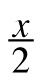+ 1 = 6 d.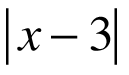+ 5 = 11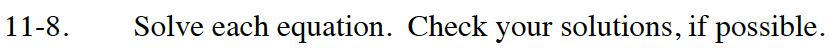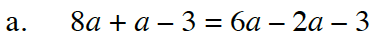Isolate the variable on one side of the equation, with the integers on the other side.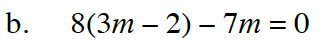Distribute before solving the equation.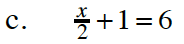Multiply each term of the equation by 2 in order to cancel out the fraction.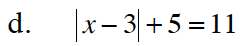There are two solutions to this equation. Why?